• +91 9971497814
• info@interviewmaterial.com

# RD Chapter 18- Surface Areas and Volume of a Cuboid and Cube Ex-VSAQS Interview Questions Answers

### Related Subjects

Question 1 : If two cubes of side 6 cm are joined face to face, then find the volume of the resulting cuboid.

Side of a cube = 6 cm
Byjoining two such cubes, the length of so
formed cuboid (l) = 6 x 2 = 12 cm
Height (h) = 6 cm
Volume= lbh = 12 x 6 x 6 cm3
= 432 cm3

Question 2 : Three cubes of metal whose edges are in the ratio 3 : 4 : 5, are melted down into a single cube whose diagonals is 12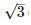cm. Find the edges of three cubes.

Answer 2 : Ratio in the sides of three cubes = 3 : 4 : 5
Let side of first cube = 3x
and side of second cube = 4x
and side of third cube = 5x
Sum of volume of threecubes
= (3x)3 + (4x)3 + (5x)3
= 27x3 + 64x3 + 125x3 = 216x3
Volume of the cube formedJby melting these three cubes = 216x3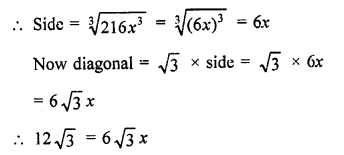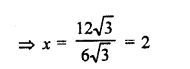Side of first cube = 3x = 3 x 2 = 6 cm
Side of second cube = 4x = 4×2 = 8 cm
and side of third cube = 5.r = 5 x 2 = 10 cm

Question 3 : If the perimeter of each face of a cube is 32 cm, find its lateral surface area. Note that four faces which meet the base of a cube are called its lateral faces.

Perimeterof each face of a cube = 32 cm
Lengthof edge = 32/4 =8 cm
and lateral surface area of the cube = 4 x (side)2
= 4 x 8 x 8 = 256 cm2

Question 4 : Find the edge of a cube whose surface area is 432 m2.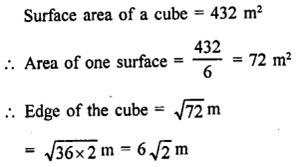Question 5 : A cuboid has total surface area of 372 cm2 and its lateral surface area is 180 cm2, find the area of its base.

Totalsurface area of a cuboid = 372 cm2
and lateral surface area = 180 cm2
Areaof base and roof = 372 – 180 = 192 cm2
and area of base =
192/2 =96 cm2

Question 6 : Three cubes of each side 4 cm are joined end to end. Find the surface area of the resulting cuboid.

By joining three cubes of side 4 cm each, end is end, we get acuboid
Length of cuboid = 4 x 3 = 12 cm
and height = 4 cm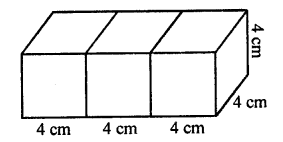Surface area = 2(lb + bh + hl)
= 2[12 x4+4×4 + 4x 12] cm2
= 2[48 + 16 + 48] cm2
= 2 x 112 = 224 cm2

Question 7 : The surface area of a cuboid is 1300 cm2. If its breadth is 10 cm and height is 20 cm, find its length.

Surfacearea of a cuboid = 1300 cm2
and height (h) = 20 cm
Let l be the length, then
= 2 (lb + bh + hl) = 1300
lb+ bh + hl =
1300/2 =650
l x 10 + 10 x 20 + 20 x l = 650
10l + 20l + 200 = 650
30l =650 – 200 = 450
l= 450/30 =15
Lengthof cuboid = 15 cm

Todays Deals### RD Chapter 18- Surface Areas and Volume of a Cuboid and Cube Ex-VSAQS Contributorskrishan

Name:
Email:

# Latest News# 9000 interview questions in different categories